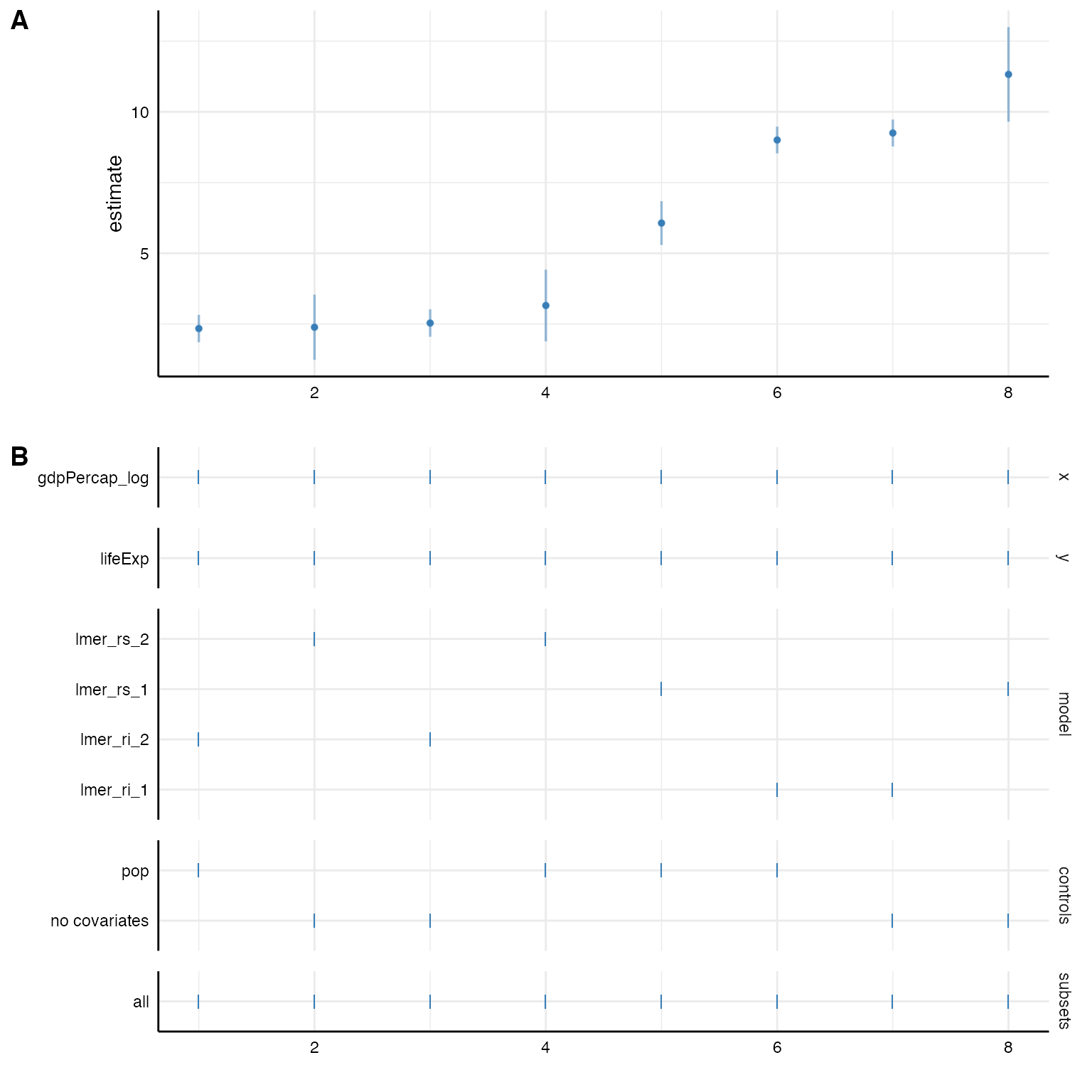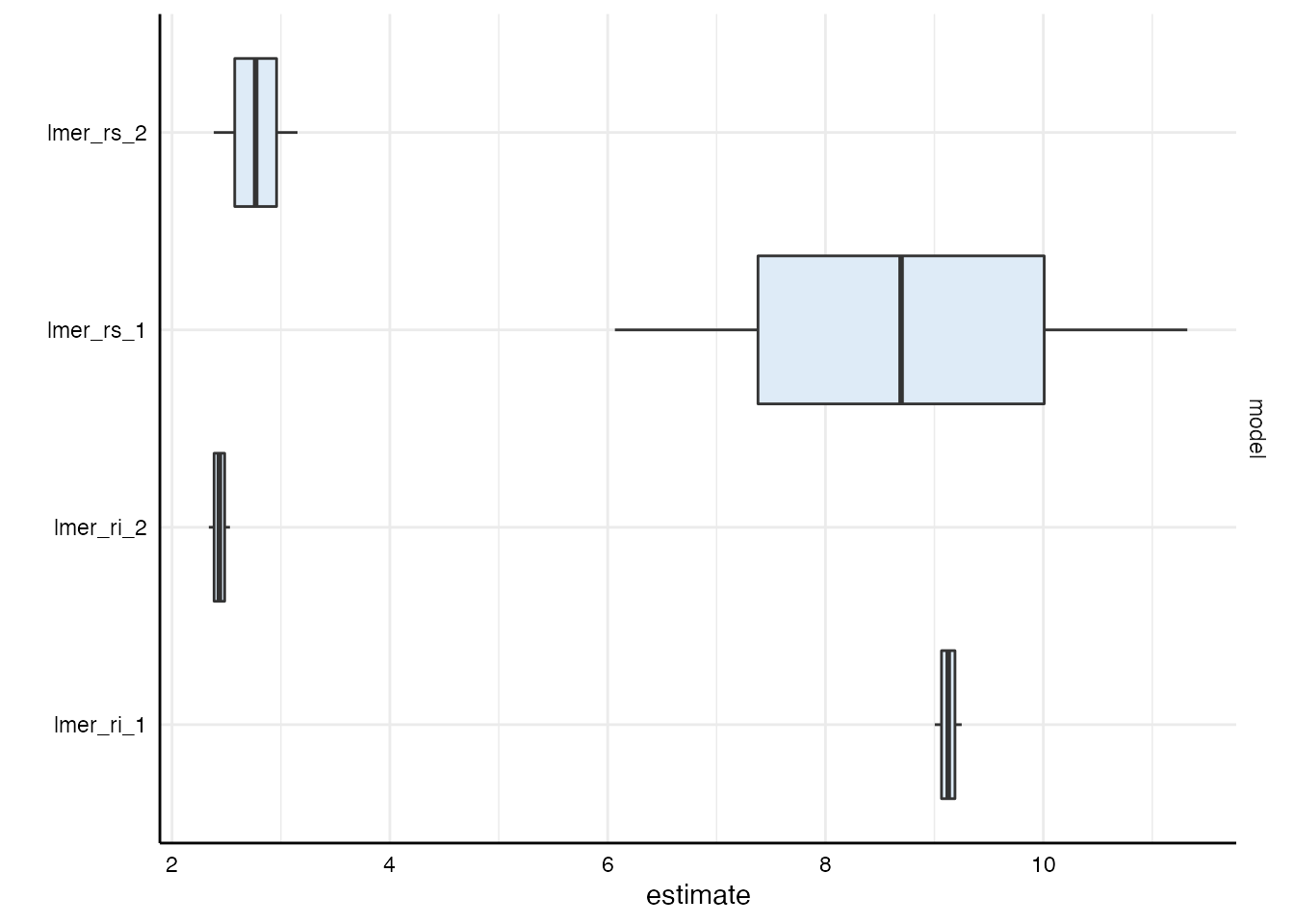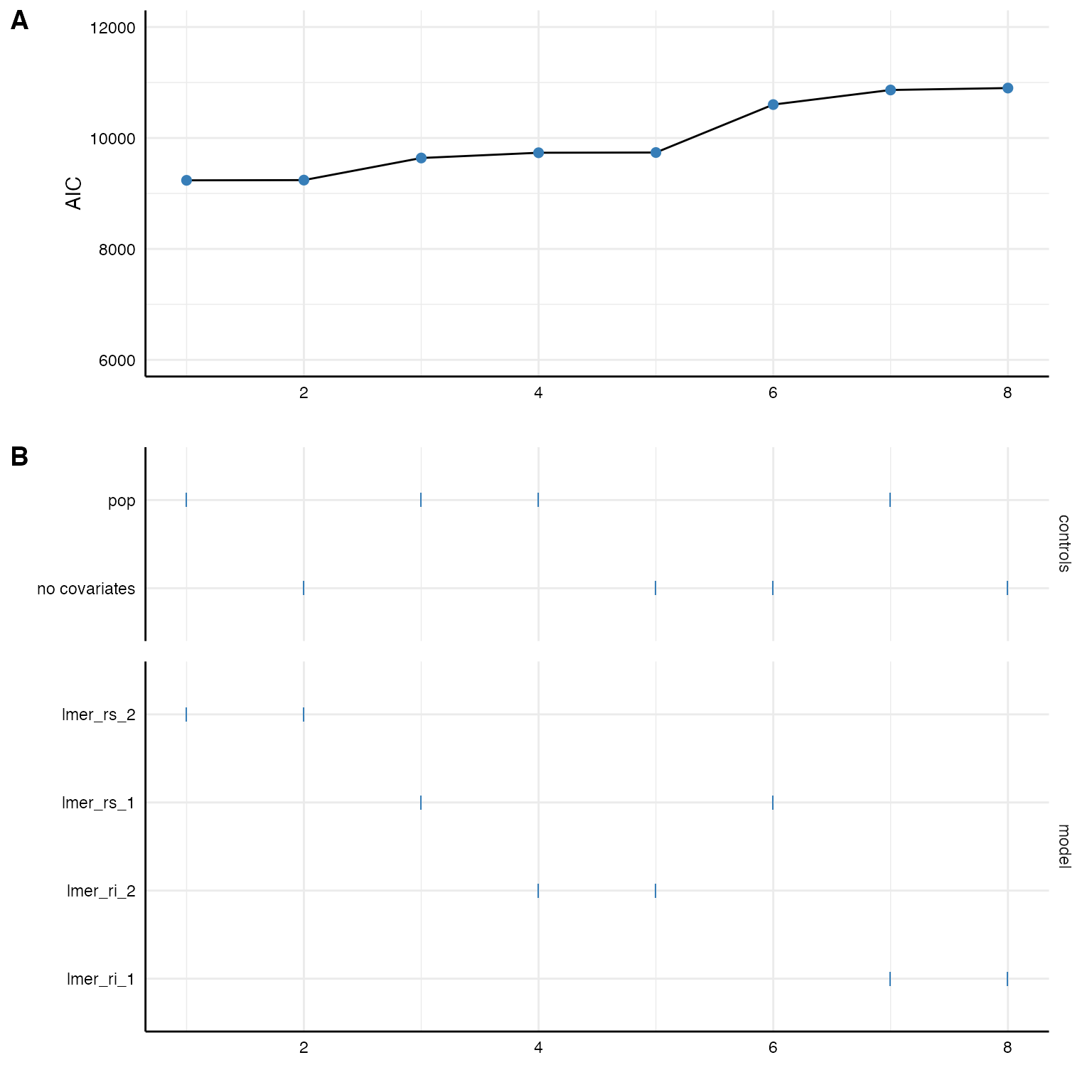Many data are hierarchical and we want to acknowledge the nested structure in our models. Specr can easily estimate such multilevel models. We again have to write a customized function and run_specs() will do the rest.

For this example, we will us the gapminder data set that is included in the gapminder-package. We quickly recode some variable to get more interpretable estiamtes

library(specr)
library(purrr)
library(dplyr)
library(ggplot2)
library(gapminder)

# Recode some variables
gapminder <- gapminder %>%
mutate(gdpPercap_log = log(gdpPercap),
pop = pop/1000)

# Check data
gapminder
#> # A tibble: 1,704 x 7
#>    country     continent  year lifeExp    pop gdpPercap gdpPercap_log
#>    <fct>       <fct>     <int>   <dbl>  <dbl>     <dbl>         <dbl>
#>  1 Afghanistan Asia       1952    28.8  8425.      779.          6.66
#>  2 Afghanistan Asia       1957    30.3  9241.      821.          6.71
#>  3 Afghanistan Asia       1962    32.0 10267.      853.          6.75
#>  4 Afghanistan Asia       1967    34.0 11538.      836.          6.73
#>  5 Afghanistan Asia       1972    36.1 13079.      740.          6.61
#>  6 Afghanistan Asia       1977    38.4 14880.      786.          6.67
#>  7 Afghanistan Asia       1982    39.9 12882.      978.          6.89
#>  8 Afghanistan Asia       1987    40.8 13868.      852.          6.75
#>  9 Afghanistan Asia       1992    41.7 16318.      649.          6.48
#> 10 Afghanistan Asia       1997    41.8 22227.      635.          6.45
#> # … with 1,694 more rows

## 1. Defining a customatized multilevel function

For this example, we use the package lme4 and more specifically the function lmer() to estimate the multilevel model (more complex models such as poisson or negative binomial multilevel models can likewise be estimated).

Based on the data set, we want to estimate the relationship between gdpPercap (GDP per capita) and lifeExp (life expectancy). Both variables are nested within both countries and years. We create several customized lmer-functions that account for different nesting strucrures.

Several aspects are important to take into account: - You need to load lavaan within the function to be able to use lmer() - You need to load broom.mixed as the standard broom::tidy function does not work for merMod-objects.

# Random intercept model (only country as grouping variable)
lmer_ri_1 <- function(formula, data,...) {
require(lme4)
require(broom.mixed)
formula <- paste(formula, "+ (1|country)")
lmer(formula, data)
}

# Including random slopes (only country as grouping variable)
lmer_rs_1 <- function(formula, data,...) {
require(lme4)
require(broom.mixed)
slopevars <- unlist(strsplit(formula, " ~ "))
formula <- paste0(formula, "+ (1 + ", slopevars, "|country)" )
lmer(formula, data)
}

# Random intercept model (lifeExp is nested in both countries and years)
lmer_ri_2 <- function(formula, data,...) {
require(lme4)
require(broom.mixed)
slopevars <- unlist(strsplit(formula, " ~ "))
formula <- paste0(formula, "+ (1|country) + (1|year)")
lmer(formula, data)
}

# Including random slopes (intercept and slopes are nested in both countries and years)
lmer_rs_2 <- function(formula, data,...) {
require(lme4)
require(broom.mixed)
slopevars <- unlist(strsplit(formula, " ~ "))
formula <- paste0(formula, "+ (1 + ", slopevars, "|country) + (", slopevars, "|year)" )
lmer(formula, data)
}

## 2. Estimate the models

We can now use these function to estimate these models. In this example, we investigate the influence of differnet nesting structure on the fixed effect between GDP per capita and life expectancy.

(results <- run_specs(df = gapminder,
y = c("lifeExp"),
x = c("gdpPercap_log"),
model = c("lmer_ri_1", "lmer_ri_2",
"lmer_rs_1", "lmer_rs_2"),
controls = "pop",
keep.results = T))
#> # A tibble: 8 x 19
#>   x     y     model controls res   effect group estimate std.error statistic
#>   <chr> <chr> <chr> <chr>    <lis> <chr>  <chr>    <dbl>     <dbl>     <dbl>
#> 1 gdpP… life… lmer… pop      <lme… fixed  <NA>      9.00     0.241     37.3
#> 2 gdpP… life… lmer… pop      <lme… fixed  <NA>      2.34     0.247      9.48
#> 3 gdpP… life… lmer… pop      <lme… fixed  <NA>      6.07     0.396     15.3
#> 4 gdpP… life… lmer… pop      <lme… fixed  <NA>      3.15     0.646      4.88
#> 5 gdpP… life… lmer… no cova… <lme… fixed  <NA>      9.25     0.242     38.2
#> 6 gdpP… life… lmer… no cova… <lme… fixed  <NA>      2.53     0.247     10.2
#> 7 gdpP… life… lmer… no cova… <lme… fixed  <NA>     11.3      0.855     13.2
#> 8 gdpP… life… lmer… no cova… <lme… fixed  <NA>      2.38     0.588      4.05
#> # … with 9 more variables: conf.low <dbl>, conf.high <dbl>, fit_sigma <dbl>,
#> #   fit_logLik <dbl>, fit_AIC <dbl>, fit_BIC <dbl>, fit_REMLcrit <dbl>,
#> #   fit_df.residual <int>, subsets <chr>

Like always, we can now inspect the results and plot the specification curve. Unfortunately, the broom.mixed::glance() does not extract the number of observations automatically. If we want to use the summarise_specs()-function, we thus quickly need to add the observations manually.

# Inspect results
results %>%
mutate(fit_nobs = map_int(res, nobs)) %>%
summarise_specs(y, model, subsets)
#> # A tibble: 4 x 10
#> # Groups:   y, model 
#>   y       model     subsets median   mad   min   max   q25   q75   obs
#>   <chr>   <chr>     <chr>    <dbl> <dbl> <dbl> <dbl> <dbl> <dbl> <dbl>
#> 1 lifeExp lmer_ri_1 all       9.13 0.183  9.00  9.25  9.07  9.19  1704
#> 2 lifeExp lmer_ri_2 all       2.43 0.144  2.34  2.53  2.39  2.48  1704
#> 3 lifeExp lmer_rs_1 all       8.69 3.90   6.07 11.3   7.38 10.0   1704
#> 4 lifeExp lmer_rs_2 all       2.77 0.570  2.38  3.15  2.58  2.96  1704

# Plot
plot_specs(results)## 3. More specific analyses

Bear in mind that run_specs() has created entire merMod objects. As we have kept these objects (by keep.results = T), we can retrieve them by looking at the column “res” and e.g., produce the standard summary of lme4.

# First model
results\$res[] %>%
summary
#> Linear mixed model fit by REML ['lmerMod']
#> Formula: lifeExp ~ gdpPercap_log + pop + (1 | country)
#>    Data: data
#>
#> REML criterion at convergence: 10855.2
#>
#> Scaled residuals:
#>     Min      1Q  Median      3Q     Max
#> -3.8643 -0.4921  0.0226  0.5402  3.0994
#>
#> Random effects:
#>  Groups   Name        Variance Std.Dev.
#>  country  (Intercept) 31.17    5.583
#>  Residual             26.98    5.194
#> Number of obs: 1704, groups:  country, 142
#>
#> Fixed effects:
#>                 Estimate Std. Error t value
#> (Intercept)   -1.470e+01  2.016e+00  -7.294
#> gdpPercap_log  9.003e+00  2.411e-01  37.345
#> pop            2.442e-05  3.117e-06   7.833
#>
#> Correlation of Fixed Effects:
#>             (Intr) gdpPr_
#> gdpPercp_lg -0.970
#> pop          0.087 -0.136
#> fit warnings:
#> Some predictor variables are on very different scales: consider rescaling

# Fixed effects for all models
results %>%
pull(res) %>%
map(fixef)
#> []
#>   (Intercept) gdpPercap_log           pop
#> -1.470391e+01  9.003243e+00  2.441721e-05
#>
#> []
#>   (Intercept) gdpPercap_log           pop
#>  3.997514e+01  2.337900e+00  1.435283e-05
#>
#> []
#>   (Intercept) gdpPercap_log           pop
#>  -0.354560951   6.066384127   0.002707258
#>
#> []
#>   (Intercept) gdpPercap_log           pop
#>  27.289551863   3.152184695   0.001445719
#>
#> []
#>   (Intercept) gdpPercap_log
#>    -15.999649      9.250647
#>
#> []
#>   (Intercept) gdpPercap_log
#>     38.817615      2.531849
#>
#> []
#>   (Intercept) gdpPercap_log
#>     -32.05618      11.32202
#>
#> []
#>   (Intercept) gdpPercap_log
#>      42.67310       2.38358

To spot difference between models, we can also use the plot_summary() function.

plot_summary(results, choices = "model")Finally, we can investigate differences in the models by comparing fit indices (e.g., AIC, BIC, deviance…).

# Create curve plot
p1 <- plot_curve(results, fit_AIC, ci = F) +
geom_line(aes(x = specifications, y = fit_AIC, color = "black")) +
geom_point(size = 2) + # increasing size of points
labs(y = "AIC")+
ylim(6000, 12000)

# Create choice panel with chisq arrangement
p2 <- plot_choices(results, fit_AIC, choices = c("controls", "model"))

# Bind together
plot_specs(plot_a = p1,
plot_b = p2)